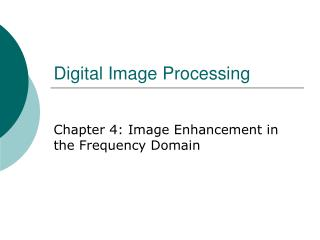DownloadDownload PresentationDigital Image Processing

# Digital Image Processing

Télécharger la présentation## Digital Image Processing

- - - - - - - - - - - - - - - - - - - - - - - - - - - E N D - - - - - - - - - - - - - - - - - - - - - - - - - - -
##### Presentation Transcript

1. Digital Image Processing Chapter 4: Image Enhancement in the Frequency Domain

2. Background • The French mathematiian Jean Baptiste Joseph Fourier • Born in 1768 • Published Fourier series in 1822 • Fourier’s ideas were met with skepticism • Fourier series • Any periodical function can be expressed as the sum of sines and/or cosines of different frequencies, each multiplied by a different coefficient

3. Fourier transform • Functions can be expressed as the integral of sines and/or cosines multiplied by a weighting function • Functions expressed in either a Fourier series or transform can be reconstructed completely via an inverse process with no loss of information

4. Applications • Heat diffusion • Fast Fourier transform (FFT) developed in the late 1950s

5. Introduction to the Fourier Transform and the Frequency Domain • The one-dimensional Fourier transform and its inverse • Fourier transform • Inverse Fourier transform

6. Two variables • Fourier transform • Inverse Fourier transform

7. Discrete Fourier transform (DFT) • Original variable • Transformed variable

8. DFT • The discrete Fourier transform and its inverse always exist • f(x) is finite in the book

9. Sines and cosines

10. Time domain • Time components • Frequency domain • Frequency components

11. Fourier transform and a glass prism • Prism • Separates light into various color components, each depending on its wavelength (or frequency) content • Fourier transform • Separates a function into various components, also based on frequency content • Mathematical prism

12. Polar coordinates • Real part • Imaginary part

13. Magnitude or spectrum • Phase angle or phase spectrum • Power spectrum or spectral density

14. Samples

15. Some references • http://local.wasp.uwa.edu.au/~pbourke/other/dft/ • http://homepages.inf.ed.ac.uk/rbf/HIPR2/fourier.htm

16. Examples • test_fft.c • fft.h • fft.c • Fig4.03(a).bmp • test_fig2.bmp

17. The two-dimensional DFT and its inverse

18. Spatial, or image variables: x, y • Transform, or frequency variables: u, v

19. Magnitude or spectrum • Phase angle or phase spectrum • Power spectrum or spectral density

20. Centering • Average gray level • F(0,0) is called the dc component of the spectrum

21. Conjugate symmetric • If f(x,y) is real • Relationships between samples in the spatial and frequency domains

22. The separation of spectrum zeros in the u-direction is exactly twice the separation of zeros in the v direction

23. Filtering in the frequency domain

24. Strong edges that run approximately at +45 degree, and -45 degree • The inclination off horizontal of the long white element is related to a vertical component that is off-axis slightly to the left • The zeros in the vertical frequency component correspond to the narrow vertical span of the oxide protrusions

25. Basics of filtering in the frequency domain • 1. Multiply the input image by to center the transform • 2. Compute F(u,v) • 3. Multiply F(u,v) by a filter function H(u,v) • 4. Compute the inverse DFT • 5. Obtain the real part • 6. Multiply the result by

26. Fourier transform of the output image • zero-phase-shift filter • Real H(u,v)

27. Inverse Fourier transform of G(u,v) • The imaginary components of the inverse transform should all be zero • When the input image and the filter function are real

28. Set F(0,0) to be zero, a notch filter

29. Lowpass filter • Pass low frequencies, attenuate high frequencies • Blurring • Highpass filter • Pass high frequencies, attenuate low frequencies • Edges, noise

30. Convolution theorem

31. Impulse function of strength A

32. Gaussian filter

33. Highpass filter

34. Smoothing Frequency-Domain Filterers • Ideal lowpass filters

35. Cutoff frequency • Total image power • Portion of the total power

36. Blurring and ringing properties • Filter • Convolution • : Spatial filter • was multiplied by • Then the inverse DFT • The real part of the inverse DFT was multiplied by